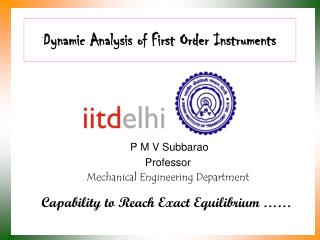# Dynamic Analysis of First Order Instruments - PowerPoint PPT PresentationDownload PresentationDynamic Analysis of First Order Instruments

Dynamic Analysis of First Order InstrumentsDownload Presentation## Dynamic Analysis of First Order Instruments

- - - - - - - - - - - - - - - - - - - - - - - - - - - E N D - - - - - - - - - - - - - - - - - - - - - - - - - - -
##### Presentation Transcript

1. Dynamic Analysis of First Order Instruments P M V Subbarao Professor Mechanical Engineering Department Capability to Reach Exact Equilibrium ……

2. First Order Instruments A first order linear instrument has an output which is given by a non-homogeneous first order linear differential equation • In these instruments there is a time delay in their response to changes of input. • The time constant t is a measure of the time delay. • Thermometers for measuring temperature are first-order instruments.

3. The time constant of a measurement of temperature is determined by the thermal capacity of the thermometer and the thermal contact between the thermometer and the body whose temperature is being measured. • A cup anemometer for measuring wind speed is also a first order instrument. • The time constant depends on the anemometer's moment of inertia.

4. First‐order Instrument Step Response b0 The complex function F(s) must be decomposed into partial fractions in order to use the tables of correspondences. This gives

5. Thermometer: A First Order Instrument Conservation of Energy during a time dt Heat in – heat out = Change in energy of thermometer Assume no losses from the stem. Heat in = Change in energy of thermometer

6. Ts(t) Ttf(t) Rs Rcond Rtf Change in energy of thermometer:

7. Step Response of Thermometers Time constant

8. Response of Thermometers: Periodic Loading If the input is a sine-wave, the output response is quite different; but again, it will be found that there is a general solution for all situations of this kind.

9. U-tube Manometer : A Second Order System • The pressure to be measured is that of a system that involves a fluid (liquid or a gas) different from the manometer liquid. • Let the density of the fluid whose pressure being measured be ρf and that of the manometer liquid be ρm. • Equilibrium of the manometer liquid requires that there be the same force in the two limbs across the plane AA. • We then have p patm This may be rearranged to read

10. Dynamic response of a U tube manometer h • The manometer liquid is assumed to be incompressible the total length of the liquid column remains fixed at L. • Assume that the manometer is initially in the equilibrium position. • The pressure difference Δp is suddenly applied across it. • The liquid column will move during time t > 0.

11. The forces that are acting on the length L of the manometer liquid are: Force disturbing the equilibrium Inertial Force Forces opposing the change: a. Weight of column of liquid b. Fluid friction due to viscosity of the liquid : • The velocity of the liquid column is expected to be small and the laminar assumption is thus valid. • The viscous force opposing the motion is calculated based on the assumption of fully developed Hagen-Poiseuelle flow. The fricitional pressure drop

12. Newton’s Law of Motion

13. Second Order System The essential parameters The static sensitivity: The dimensionless damping ratio: The Natural Frequency:

14. Transfer Function of a second order system:

15. The transfer function is parameterized in terms of ζ and ωn. • The value of ωn doesn’t qualitatively change the system response. • There are three important cases—withqualitatively different system behavior—as ζ varies. • The three cases are called: • Over Damped System (ζ >1) • Critically Damped System (ζ =1) • Under Damped System (ζ <1)

16. Over Damped System (ζ >1)

17. y(t) t

18. General Response of A Second Order System t z=0 y(t) t z=0.5

19. z=0.707 z=1.0 t

20. Response of U tube manometer to step input